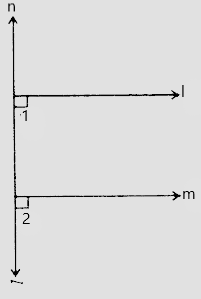# If $l, m, n$ are three lines such that $l \parallel m$ and $n \perp l$, prove that $n \perp m$.

Given:

$l, m, n$ are three lines such that $l \parallel m$ and $n \perp l$.

To do:

We have to prove that $n \perp m$.

Solution:$n \perp l$

This implies,

$\angle 1 = 90^o$

$l \parallel m$ and $n$ is the transversal.

Therefore,

$\angle l = \angle 2$                         (Corresponding angles are equal)

$\angle 2 = 90^o$

This implies,

$n \perp m$.

Hence proved.

Updated on: 10-Oct-2022

38 Views